This tutorial is based on essential background material described in the soilDB and related NASIS ODBC connection tutorials. If you have not reviewed those tutorials, please do so before proceeding.

The functions and documentation described in this tutorial are still a work in progress.

# Introduction

The `soilDB` package contains a number of convenience functions (usually having a prefix of "fetch") for extracting data from commonly used databases, performing basic quality control checks, and returning the result as a SoilProfileCollection object. In order to simplify the process of stitching-together the many linked (and sometimes nested) tables associated with data in NASIS, convenience functions (e.g. `fetchNASIS()`) must make a number of assumptions about the data. This document describes how those assumptions can be adjusted and how they may affect subsequent data analysis.

# Component Data

Load up your local database and selected set with some components. NASIS queries should "target" the tables:

• Component
• Data Mapunit
• Correlation
• Mapunit
• Legend Mapunit
• Legend
• Area

The most commonly used data from the data map unit, component, component horizon tables can be quickly loaded using the `fetchNASIS()` function.

``````# load required libraries
library(soilDB)
library(ggplot2)
library(plyr)

# get component + component horizon data as a SoilProfileCollection, using defaults
nc <- fetchNASIS(from = 'components')``````

The result is a SoilProfileCollection object and can be subset or visualized as such. Lets try extracting just the components with names that match the pattern "Amador".

``````# filter out just those components named "Amador"
idx <- grep('Amador', nc\$compname, ignore.case = TRUE)
nc.sub <- nc[idx, ]

# plot
par(mar=c(2,0,3,0))
plot(nc.sub, color='claytotal_r', label='dmudesc')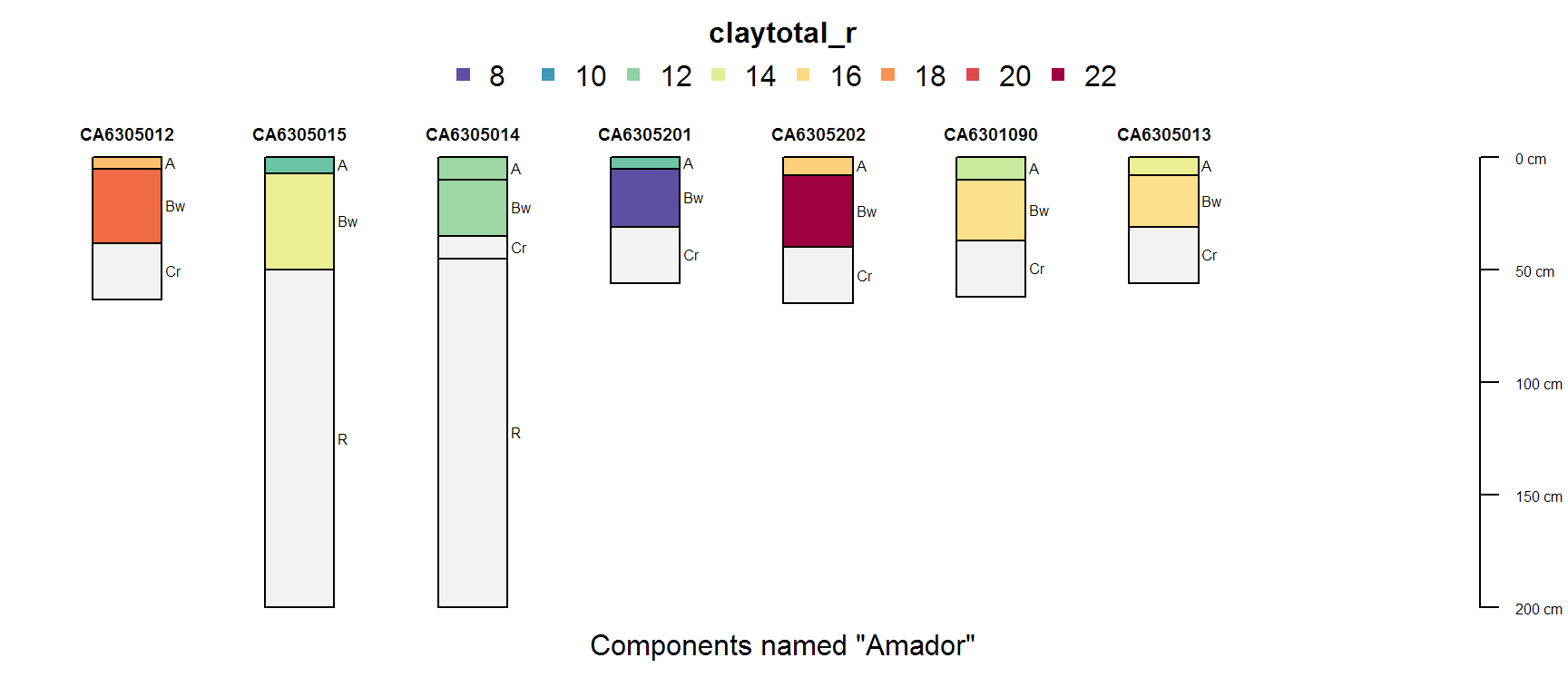There appear to be many duplicate components. This isn't surprising as a single component template is commonly shared among many map units. Lets filter out a unique subset of components based on horizon depth, horizon designation, and clay content.

``````# generate an index to unique components and subset
idx <- unique(nc.sub, vars=c('hzdept_r', 'hzdepb_r', 'claytotal_r', 'hzname'))
nc.sub.unique <- nc.sub[idx, ]

# plot the subset
par(mar=c(2,0,3,0))
plot(nc.sub.unique, color='claytotal_r', label='dmudesc', n=length(nc.sub))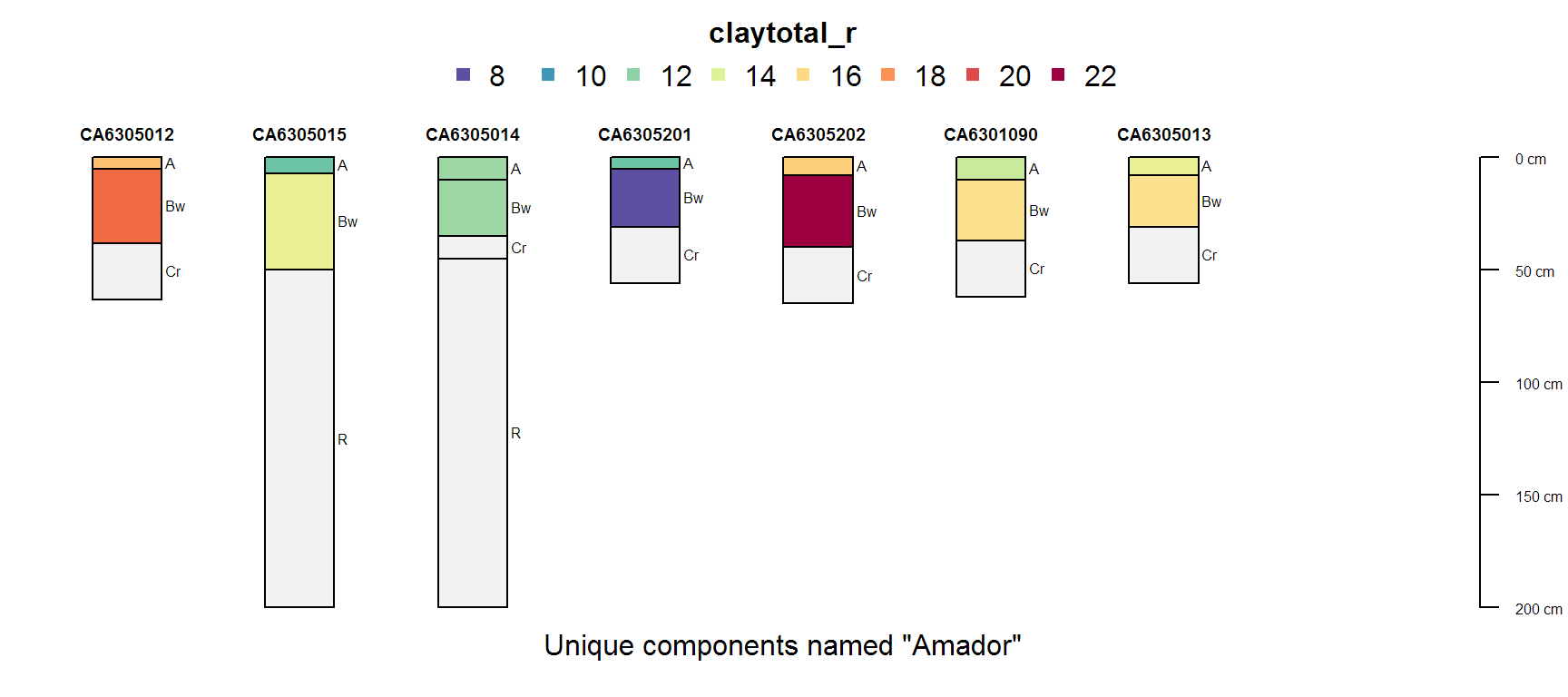# Accessing Additional Types of "Component" Data

Sometimes it is convenient to know which pedons are linked to components. The following function returns a `data.frame` (table) of data containing component record IDs, pedon record IDs, and pedon IDs. Lets assemble a list of all pedons linked to a component named "Amador", using the subset from above.

``````# get the full set of linked pedon data from the selected set
# check format
``````##     coiid   peiid      pedon_id representative
## 1 2073965  207247      06JCR002              1
## 2 2076762  530760      11BJM046              1
## 3 2045840  620506      11DEB041              0
## 4 2528931  839210 2012CA6302054              1
## 5 2278328  978613 2014CA6304027              1
## 6 2528901 1209611 2017CA6303009              1``````
``````# list just those pedons that are linked to our subset of components
# note that we are using the component record ID (coiid) to connect the two data sources
``````##       coiid   peiid      pedon_id representative
## 1   2073965  207247      06JCR002              1
## 4   2528931  839210 2012CA6302054              1
## 5   2278328  978613 2014CA6304027              1
## 6   2528901 1209611 2017CA6303009              1
## 960 2331930  620494  S2011DEB094N              1
## 966 2331929  978594 2013CA6304020              1``````

## Map Unit, Correlation, Legend, Area Tables

The NASIS data elements that exist at levels higher than the data map unit table must be loaded with a different function because there isn't always a 1:1 mapping between data map unit objects and map unit / legend objects.

``````nc.correlation <- get_component_correlation_data_from_NASIS_db()
``````##     muiid musym nationalmusym
## 3 1865918  3046         20mmx
##                                                                             muname  mukind mutype
## 3 Goldwall, intermound-Goldwall, mound-Rock outcrop complex, 0 to 8 percent slopes complex   <NA>
##      mustatus muacres farmlndcl repdmu dmuiid areasymbol
## 3 provisional    5561      <NA>      1 524297      CA630
##                                                                              areaname ssastatus
## 3 Central Sierra Foothills Area, California, Parts of Calaveras and Tuolumne Counties   initial
##   cordate
## 3    <NA>``````

Lets tabulate the occurrence of components named "Amador" by soil survey area and component kind, using data loaded in the above examples.

``````# combine basic site data + correlation / legend data
# note that there is a 1:many relationship
nc.combined <- join(site(nc.sub), nc.correlation, by='dmuiid')

# cross-tabulate
xtabs( ~ areasymbol + compkind, data=nc.combined)``````
``````##           compkind
##      CA630      6          1``````

## Component Month Table

It can be convenient to join elements from the DMU, component, and comonth tables into a single `data.frame`. Lets start by loading the DMU/component records and creating a new ID that combines the DMU description and component name.

``````# load only the component table
comp <- get_component_data_from_NASIS_db()
# make a new ID by combining DMU name + comp name
comp\$id <- paste0(comp\$dmudesc, '-', comp\$compname)``````

The comonth records are indexed by `coiid` values. In some cases there are no records populated in this table. You can "fill" missing records with `NA` by using the `fill=TRUE` argument.

``````# load comonth as-is: there will be missing records
cm <- get_comonth_from_NASIS_db()
nrow(cm)``````
``##  6718``
``````# load comonth after filling missing months
cm <- get_comonth_from_NASIS_db(fill = TRUE)
nrow(cm)``````
``##  11231``
``````# there should be the same number of "coiid" values after filling missing comonth records
length(comp\$coiid) == length(unique(cm\$coiid))``````
``##  FALSE``
``````# join component table to "filled" comonth table
x <- join(comp, cm, by='coiid')
nrow(x)``````
``##  8556``

Try plotting some of the combined data, for select DMU and components. NULL values are displayed as grey squares.

``ggplot(subset(x, subset= dmudesc == 'CA6305015'), aes(month, factor(id), flodfreqcl)) + geom_tile(aes(fill = flodfreqcl), color='white', lwd=1) + scale_fill_brewer(palette = "Spectral", drop=FALSE, na.value='grey80', name='Flooding Frequency') + scale_x_discrete(drop=FALSE) + theme_minimal() + xlab('') + ylab('')  ``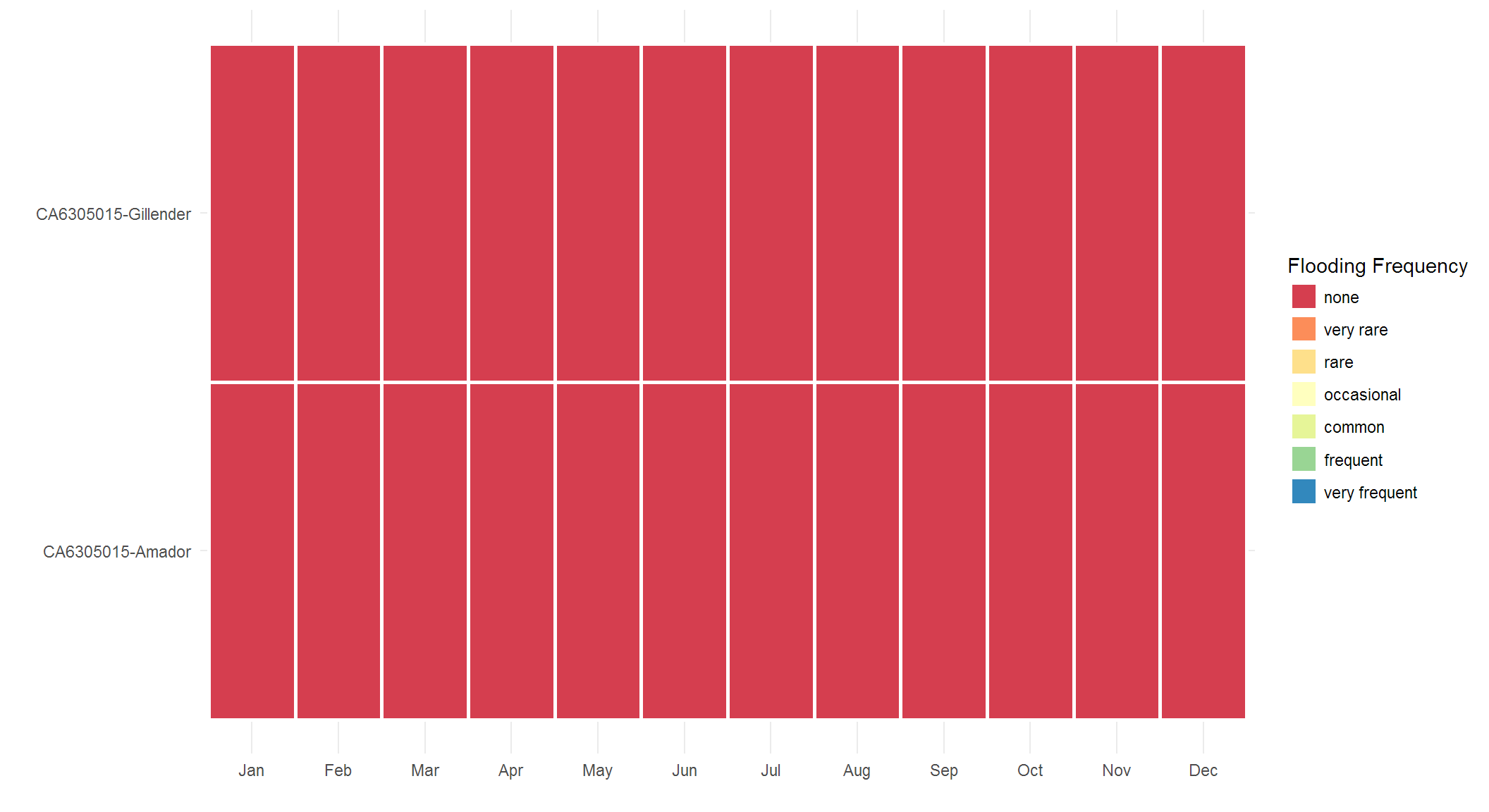``ggplot(subset(x, subset= compname == 'Gillender'), aes(month, factor(id), flodfreqcl)) + geom_tile(aes(fill = flodfreqcl), color='white', lwd=1) + scale_fill_brewer(palette = "Spectral", drop=FALSE, na.value='grey80', name='Flooding Frequency') + scale_x_discrete(drop=FALSE) + theme_minimal() + xlab('') + ylab('')  ``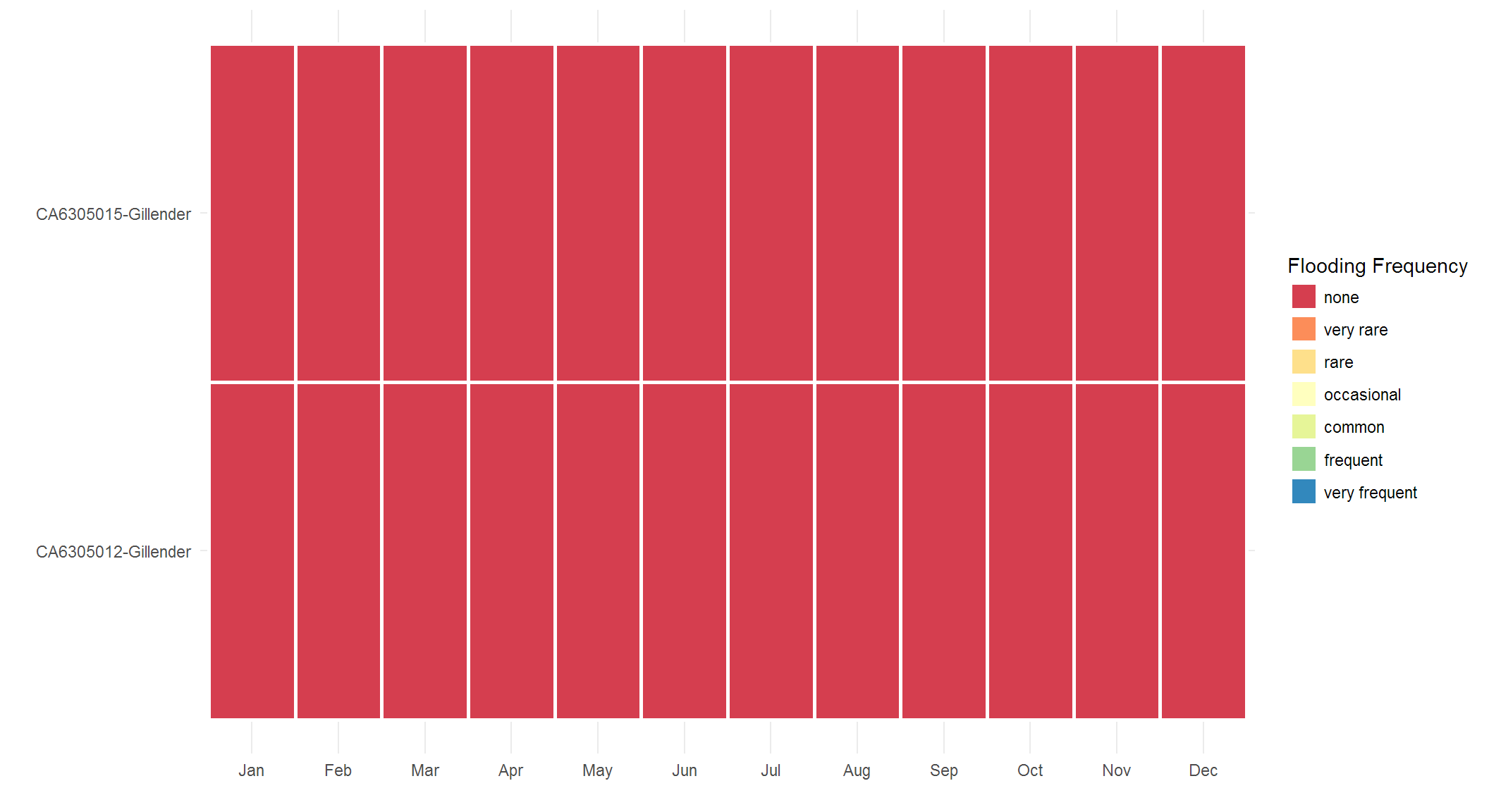Try plotting those components with flooding / ponding frequency classes that are something other than "None" (or NULL).

``````idx <- which(x\$flodfreqcl != 'none')
ggplot(subset(x, subset= coiid %in% x\$coiid[idx]), aes(month, factor(id), flodfreqcl)) + geom_tile(aes(fill = flodfreqcl), color='white', lwd=1) + scale_fill_brewer(palette = "Spectral", drop=FALSE, na.value='grey80', name='Flooding Frequency') + scale_x_discrete(drop=FALSE) + theme_minimal() + xlab('') + ylab('')``````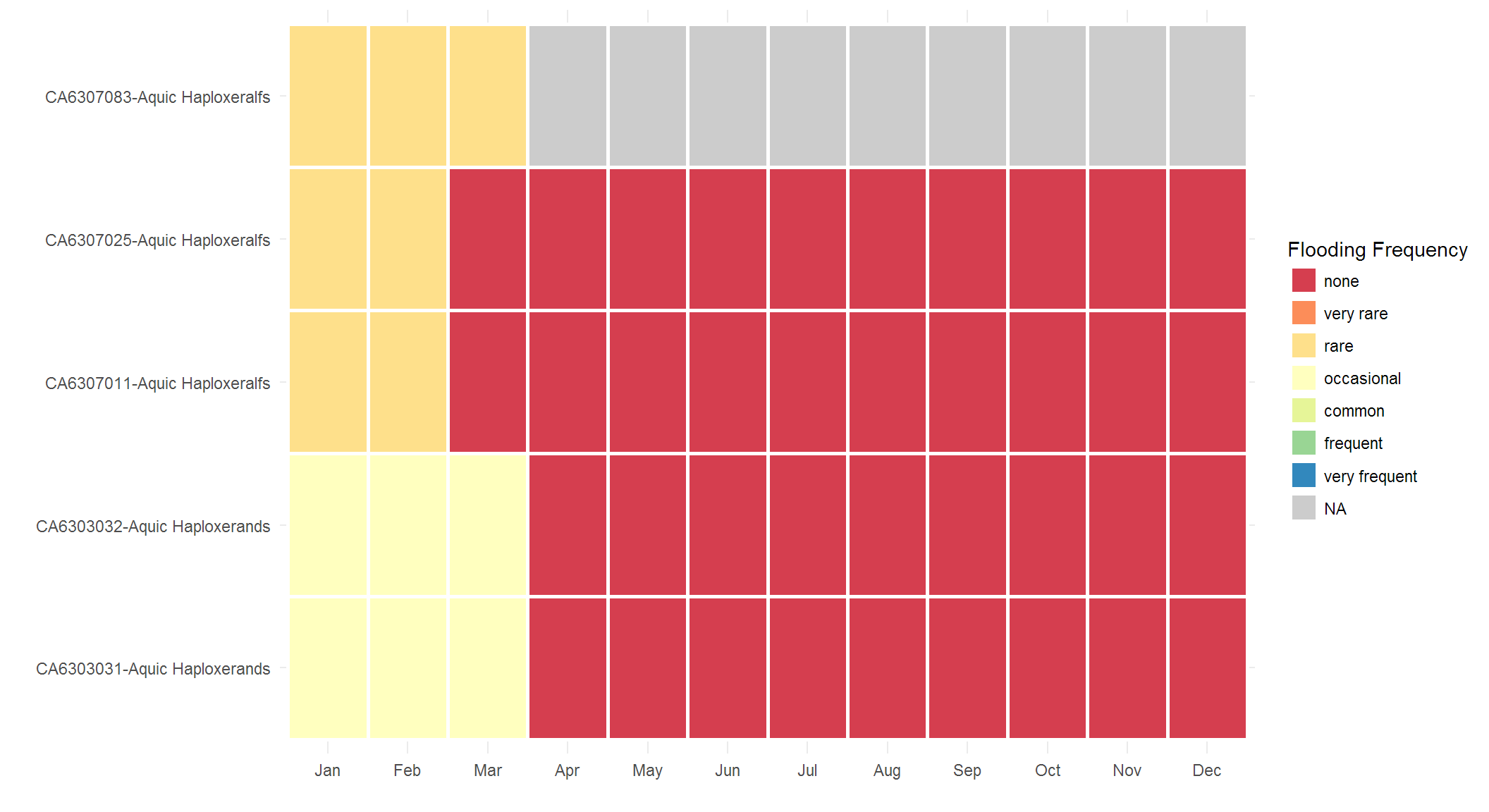``````idx <- which(x\$floddurcl != 'none')
ggplot(subset(x, subset= coiid %in% x\$coiid[idx]), aes(month, factor(id), floddurcl)) + geom_tile(aes(fill = floddurcl), color='white', lwd=1) + scale_fill_brewer(palette = "Spectral", drop=FALSE, na.value='grey80', name='Flooding Duration') + scale_x_discrete(drop=FALSE) + theme_minimal() + xlab('') + ylab('')``````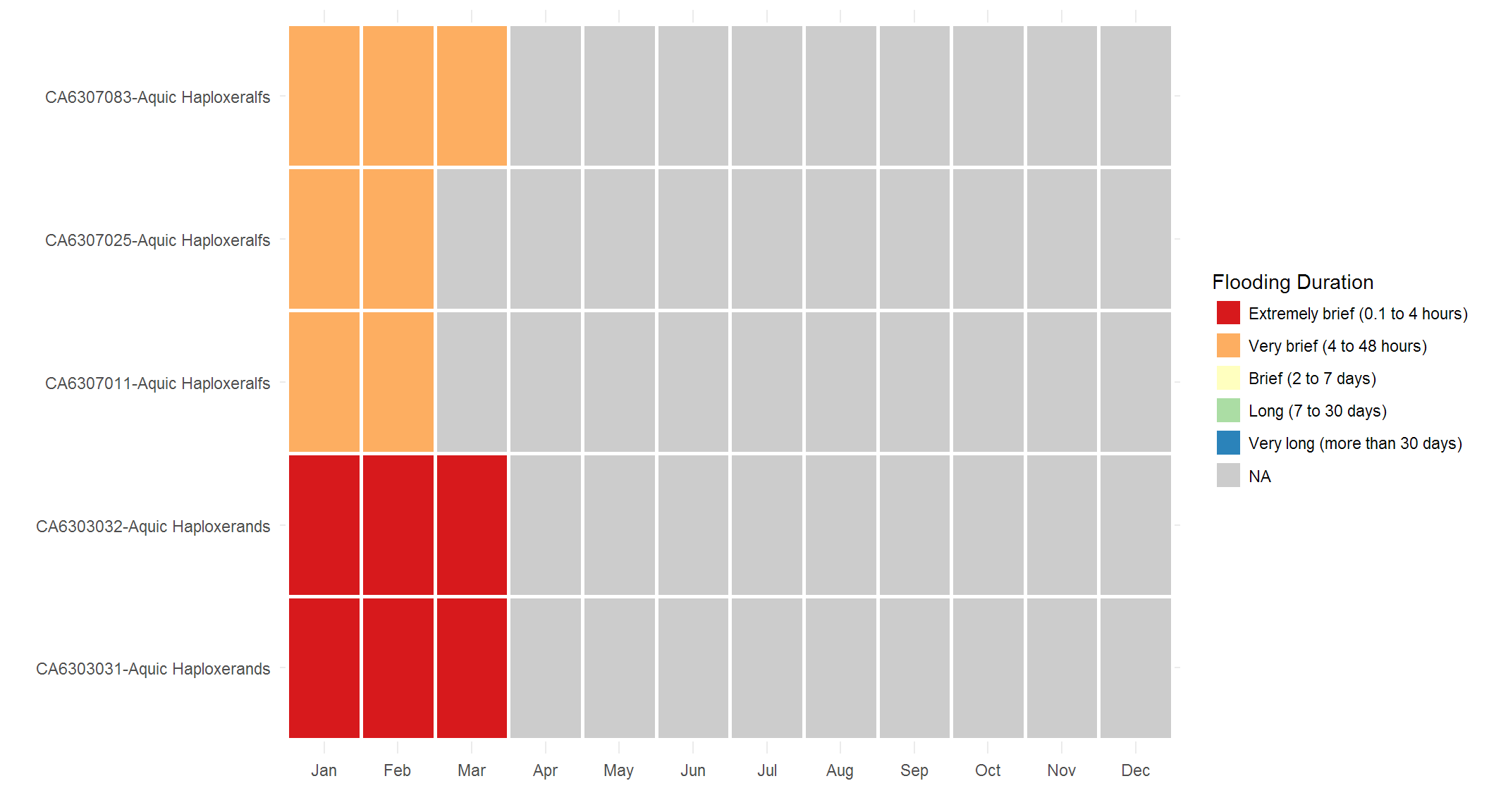``````idx <- which(x\$pondfreqcl != 'none')
ggplot(subset(x, subset=coiid %in% x\$coiid[idx]), aes(month, factor(id), pondfreqcl)) + geom_tile(aes(fill = pondfreqcl), color='white', lwd=1) + scale_fill_brewer(palette = "Spectral", drop=FALSE, na.value='grey80', name='Ponding Frequency') + scale_x_discrete(drop=FALSE) + theme_minimal() + xlab('') + ylab('')``````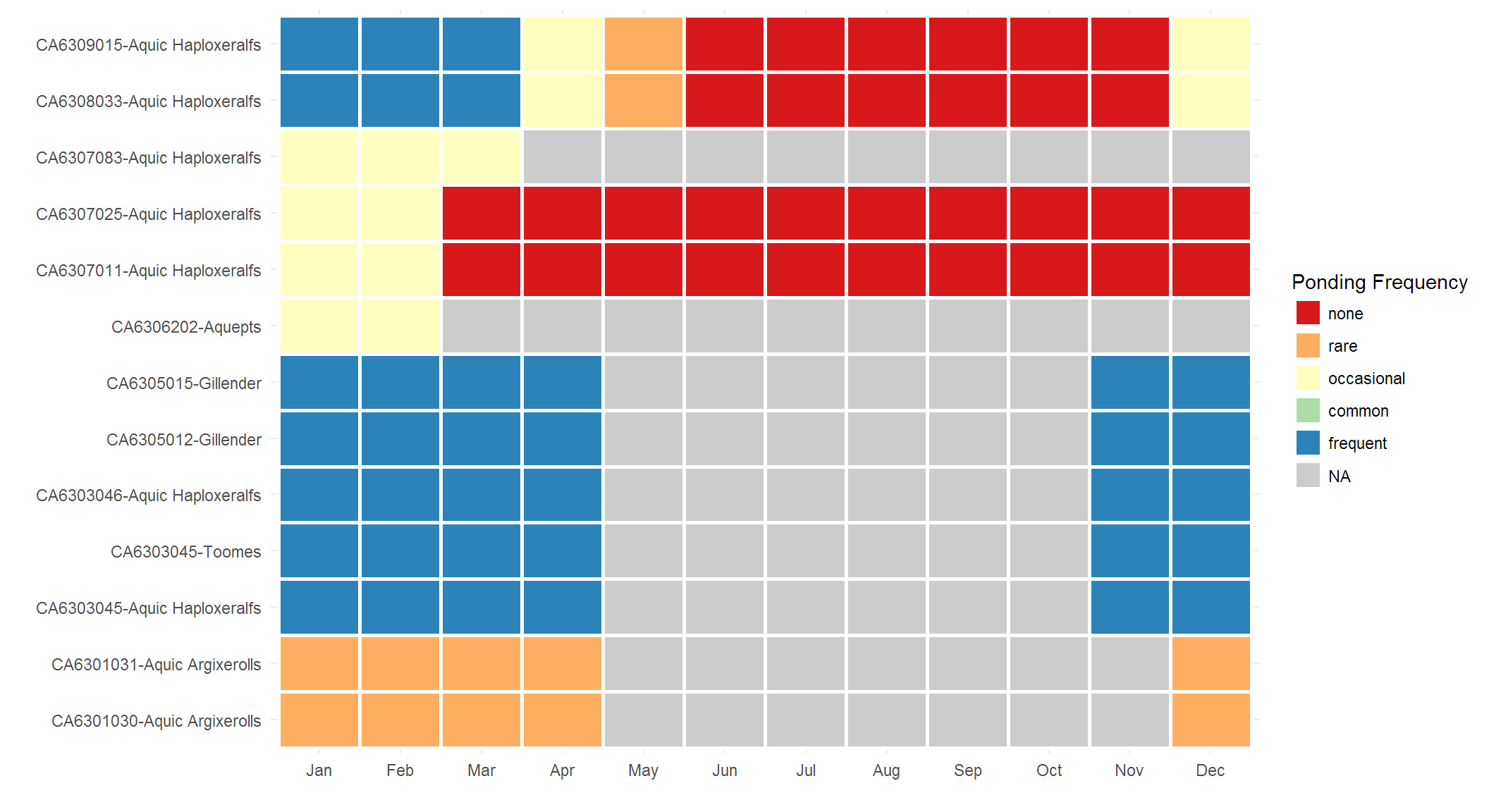``````idx <- which(x\$ponddurcl != 'none')
ggplot(subset(x, subset= coiid %in% x\$coiid[idx]), aes(month, factor(id), ponddurcl)) + geom_tile(aes(fill = ponddurcl), color='white', lwd=1) + scale_fill_brewer(palette = "Spectral", drop=FALSE, na.value='grey80', name='Ponding Duration') + scale_x_discrete(drop=FALSE) + theme_minimal() + xlab('') + ylab('')``````